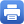Columbia Home
SPRING 2020 SAMUEL EILENBERG LECTURES

Heat Equations in Analysis, Geometry, and Probability

“In its most basic form, the heat equation is a partial differential equation that describes the evolution of temperature in space over time. This and similar equations play a central role in diverse fields. The goal is to provide an introduction to the heat equation and its generalizations, including connections to geometry and geometric flows, probability, and, of course, analysis.”

Tuesdays at 2:40 pm

Room 520, Mathematics HallPrint this page